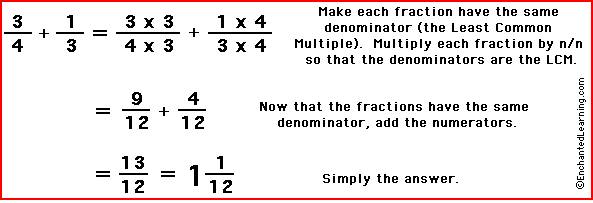# Unit 4!

``` On this page we will be talking about adding two different fractions, using
LCM to make the denominators the same. After adding them together, we then simplify. ```

## Our Fraction: 4/(x+3) + 2x/(x2-9)

### First Step: Writing out the fraction

4/(x+3) + 2x/(x2-9)

### Second Step: Finding the Least Common Denominator

Denominator 1 = X + 3 Denominator 2 = X2- 9 X2- 9 = (x-3)(x+3) LCM = (x+3)(x-3)

The first denominator can not be simplified; It has an X and a 3, attached by addition. The second denominator can be simplified. This is because the denominator is a difference of two squares. The requirements for the difference of two squares is that the fraction must have two variables, both producing whole number when finding the square root. The second requirement being that the denominator is attached by subtration. In our case, a difference of two squares is simplified down to (x+3)(x-3). From there, we're able to find the least common denominator which would be (x+3)(x-3) because we incomportated both denominators into one.

### Third Step: Multiply All Numerators By What Is Missing

4(x+3)/(x+3)(x-3) + 2x/X2- 9 4x-12/LCM + 2x/LCM

After finding the LCM, we must cross multiply the fraction by what is missing so we can add them. We're able to add them afterwards because they share the same denominator. The first step is to find what is missing from the particular fraction, then multiply both the numerator and the denominator.